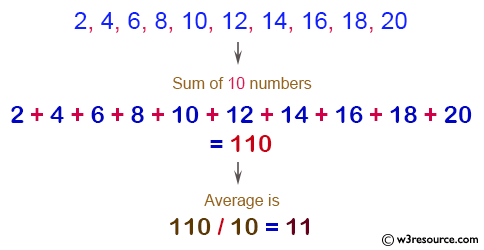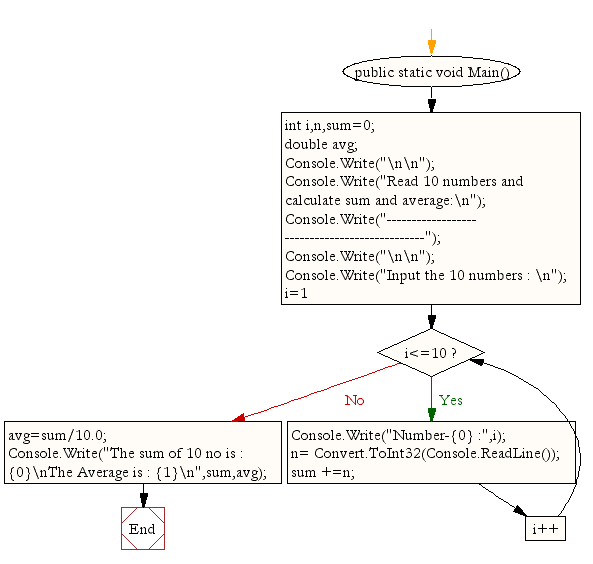﻿ C# - Read 10 numbers and find their sum and average# C# Sharp Exercises: Read 10 numbers and find their sum and average

## C# Sharp For Loop: Exercise-4 with Solution

Write a program in C# Sharp to read 10 numbers from keyboard and find their sum and average.

Pictorial Presentation:Sample Solution:

C# Sharp Code:

``````using System;
public class Exercise4
{
public static void Main()
{
int i,n,sum=0;
double avg;

Console.Write("\n\n");
Console.Write("Read 10 numbers and calculate sum and average:\n");
Console.Write("----------------------------------------------");
Console.Write("\n\n");

Console.Write("Input the 10 numbers : \n");
for (i=1;i<=10;i++)
{
Console.Write("Number-{0} :",i);

sum +=n;
}
avg=sum/10.0;
Console.Write("The sum of 10 no is : {0}\nThe Average is : {1}\n",sum,avg);
}
}
```
```

Sample Output:

```Read 10 numbers and calculate sum and average:
----------------------------------------------

Input the 10 numbers :
Number-1 :2
Number-2 :4
Number-3 :6
Number-4 :8
Number-5 :10
Number-6 :12
Number-7 :14
Number-8 :16
Number-9 :18
Number-10 :20
The sum of 10 no is : 110
The Average is : 11
```

Flowchart:C# Sharp Code Editor:

Contribute your code and comments through Disqus.

What is the difficulty level of this exercise?

Test your Programming skills with w3resource's quiz.

﻿